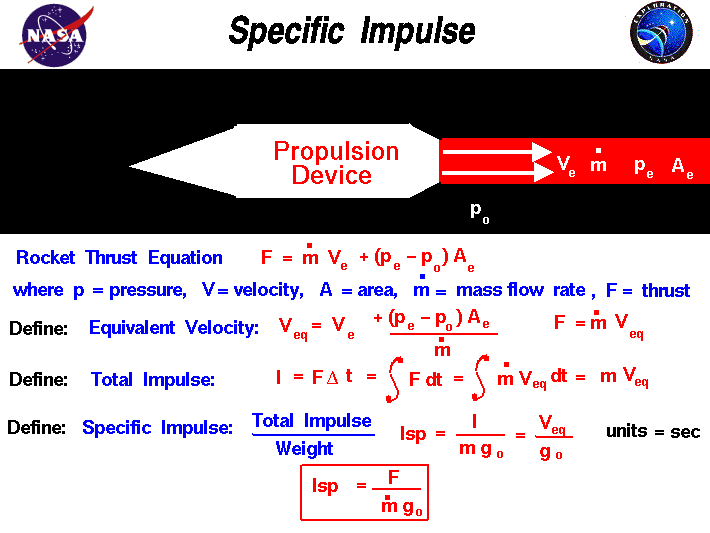+ Text Only Site
+ Non-Flash Version
+ Contact GlennThrust is the force which moves a rocket through the air. Thrust is generated by the rocket engine through the reaction of accelerating a mass of gas. The gas is accelerated to the the rear and the rocket is accelerated in the opposite direction. To accelerate the gas, we need some kind of propulsion system. We will discuss the details of the propulsion system on some other pages. For right now, let us just think of the propulsion system as some machine which accelerates a gas. From Newton's second law of motion, we can define a force to be the change in momentum of an object with a change in time. Momentum is the object's mass times the velocity. When dealing with a gas, the basic thrust equation is given as: F = mdot e * Ve - mdot 0 * V0 + (pe - p0) * Ae Thrust F is equal to the exit mass flow rate mdot e times the exit velocity Ve minus the free stream mass flow rate mdot 0 times the free stream velocity V0 plus the pressure difference across the engine pe - p0 times the engine area Ae. For liquid or solid rocket engines, the propellants, fuel and oxidizer, are carried on board. There is no free stream air brought into the propulsion system, so the thrust equation simplifies to: F = mdot * Ve + (pe - p0) * Ae where we have dropped the exit designation on the mass flow rate. Using algebra, let us divide by mdot: F / modt = Ve + (pe - p0) * Ae / mdot We define a new velocity called the equivalent velocity Veq to be the velocity on the right hand side of the above equation: Veq = Ve + (pe - p0) * Ae / mdot Then the rocket thrust equation becomes: F = mdot * Veq The total impulse (I) of a rocket is defined as the average thrust times the total time of firing. On the slide we show the total time as "delta t". (delta is the Greek symbol that looks like a triangle): I = F * delta t Since the thrust may change with time, we can also define an integral equation for the total impulse. Using the symbol (Sdt) for the integral, we have: I = S F dt Substituting the equation for thrust given above: I = S (mdot * Veq) dt Remember that mdot is the mass flow rate; it is the amount of exhaust mass per time that comes out of the rocket. Assuming the equivalent velocity remains constant with time, we can integrate the equation to get: I = m * Veq where m is the total mass of the propellant. We can divide this equation by the weight of the propellants to define the specific impulse. The word "specific" just means "divided by weight". The specific impulse Isp is given by: Isp = Veq / g0 where g0 is the gravitational acceleration constant (32.2 ft/sec^2 in English units, 9.8 m/sec^2 in metric units). Now, if we substitute for the equivalent velocity in terms of the thrust: Isp = F / (mdot * g0) Mathematically, the Isp is a ratio of the thrust produced to the weight flow of the propellants. A quick check of the units for Isp shows that: Isp = m/sec / m/sec^2 = sec Why are we interested in specific impulse? First, it gives us a quick way to determine the thrust of a rocket, if we know the weight flow rate through the nozzle. Second, it is an indication of engine efficiency. Two different rocket engines have different values of specific impulse. The engine with the higher value of specific impulse is more efficient because it produces more thrust for the same amount of propellant. Third, it simplifies our mathematical analysis of rocket thermodynamics. The units of specific impulse are the same whether we use English units or metric units. Fourth, it gives us an easy way to "size" an engine during preliminary analysis. The result of our thermodynamic analysis is a certain value of specific impulse. The rocket weight will define the required value of thrust. Dividing the thrust required by the specific impulse will tell us how much weight flow of propellants our engine must produce. This information determines the physical size of the engine. Guided ToursRocket Thrust:Model Rocket:Scalars:Rocket Thrust Simulator:Activities: Related Sites: Rocket Index Rocket Home Beginner's Guide Home+ Inspector General Hotline + Equal Employment Opportunity Data Posted Pursuant to the No Fear Act + Budgets, Strategic Plans and Accountability Reports + Freedom of Information Act + The President's Management Agenda + NASA Privacy Statement, Disclaimer, and Accessibility CertificationEditor: Tom Benson NASA Official: Tom Benson Last Updated: May 13 2021 + Contact Glenn